## 1. Introduction

In this tutorial, we’ll study bit shift operators.

## 2. Bit Shift Operators

A bit shift operator is a low-level operator that works on the individual bits of an integer.

It takes two operands. One is the integer whose bits we want to shift. The other represents the number of shifts. The result of a bit shift is a new integer.

Bit-shift operators are easy to use and execute quickly on modern multicore CPU systems. We use them to reduce memory usage and speed up execution.

### 2.1. Classification

Shifts can be left or right. The left ones shift the bits to the left, and the right ones shift them to the right. If they discard the bits in the direction of shifting, we call them non-circular.

There are also circular shift operations. For example, in the circular left shift, we move the most significant bit (MSB) to the position of the least significant bit (LSB) as we shift all the bits to their left. We do a similar thing in the circular right shift. However, almost all programming languages (C, C++, Java) lack native operators or built-in functions for circular shifting.

## 3. Left Shift

In this section, we focus on the non-circular left shift operator (<<). It shifts the first operand to the left by the number of bits specified by the second operand.

For example,will give us 22: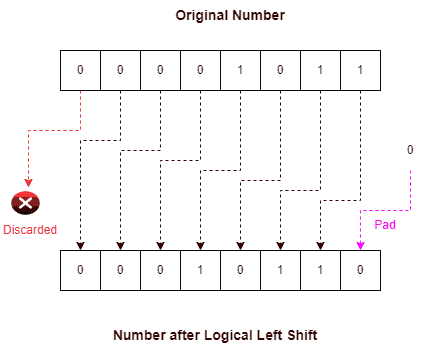Internally, this operation shifts all the bits to the left and fills the vacant places with zeros.

The left shift operator implements multiplication by 2. Most modern compilers (including GCC and CLANG) replace all multiplication by 2 (and its powers) with equivalent left bit-shift operations.

## 4. Right Shift

As the name suggests, the right shift operator (>> or >>>) shifts the first operand by the same number of bits to the right as specified by the second operand. In the logical right shift, we fill the vacant places with zeros; in the arithmetic right shift, we fill them with the most significant digit (the sign bit).

Some programming languages, such as C and C++, can apply the right shift operator (>>) only to signed integer types. In contrast, in other languages such as Java or C#, the right shift behaves differently for signed and unsigned types.

### 4.1. Logical Right Shift

In the logical right shift (>>>), we shift our number by a given number of bits to the right and later pad the vacant places with zeros. For example,will give us 5: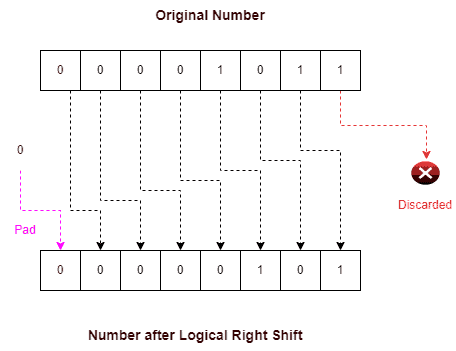So, a logical right shift by one bit is equivalent to the integer division by 2 ().

This operation results in a loss of information since we can’t recover the overwritten bits.

For example, right-shiftingby two bits followed by two left bit-shifts doesn’t give usbut.

### 4.2. Arithmetic Right Shift

The arithmetic right shift (>>) is similar to the logical right shift but with one difference. Here, we don’t pad with zeros but the most significant bit (MSB).

If the integer is signed, MSB represents its sign. Hence, the arithmetic right shift preserves the sign: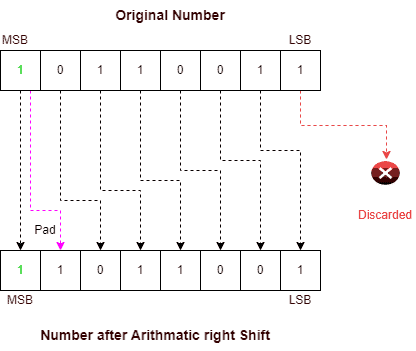We understand it better with an example. Letbe stored in its 2’s complement form as.

Now, we do an arithmetic right shift by one place and getin 2’s complement. The result is -8 in the decimal system.

So, the right arithmetic shift divides a negative integer (represented by 2’s complement) by two while preserving its sign.

## 5. Circular Shift

Now, let’s discuss circular shifting.

We use circular shifts while implementing cryptographic algorithms. Unfortunately, these shifts don’t have native support in most programming languages. So, we have to implement them using built-in bit operators.

In a circular shift, we don’t discard any bit or pad the vacant places with 0 or the sign bit. Instead, we circularly rotate the bits per the shift’s direction. Hence, depending on direction, we have the left and right circular shifts.

### 5.1. Left Circular Shift

In the left circular shift, we move all bits to the left, filling the vacant places on the right with digits replaced from the left in the same order. So, the old MSB becomes the new LSB. For example, circular left shifting oftwice will give us 14: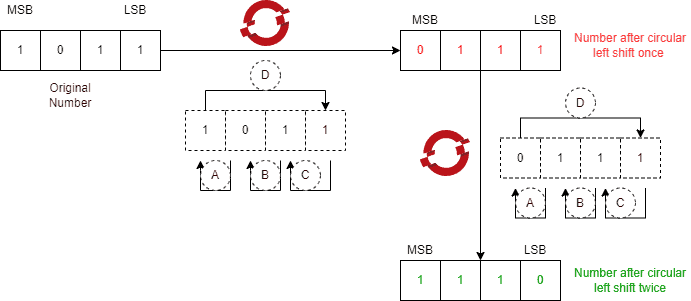In contrast to the logical left shift, the circular left shift doesn’t multiply by a power of two.

### 5.2. Right Circular Shift

In the right circular shift, we move all bits shift to the right, with the bit at the LSB location rotating back to the MSB location. For example, circular right shifting oftwice will give us 14: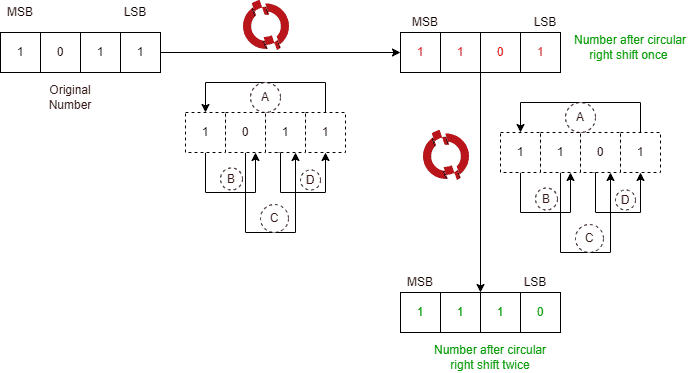In contrast to the logical right shift, the circular right shift doesn’t divide the integer by a power of two.

Also, circular left and right shifts don’t lose original bits and are inverse to one another.

## 6. Conclusion

In this article, we’ve studied bit shift operators in detail. All modern compilers optimize our code by substituting bit shifts for multiplications and divisions. This is so because bit shift operations are much faster to map onto the low-level CPU operations of a CPU.